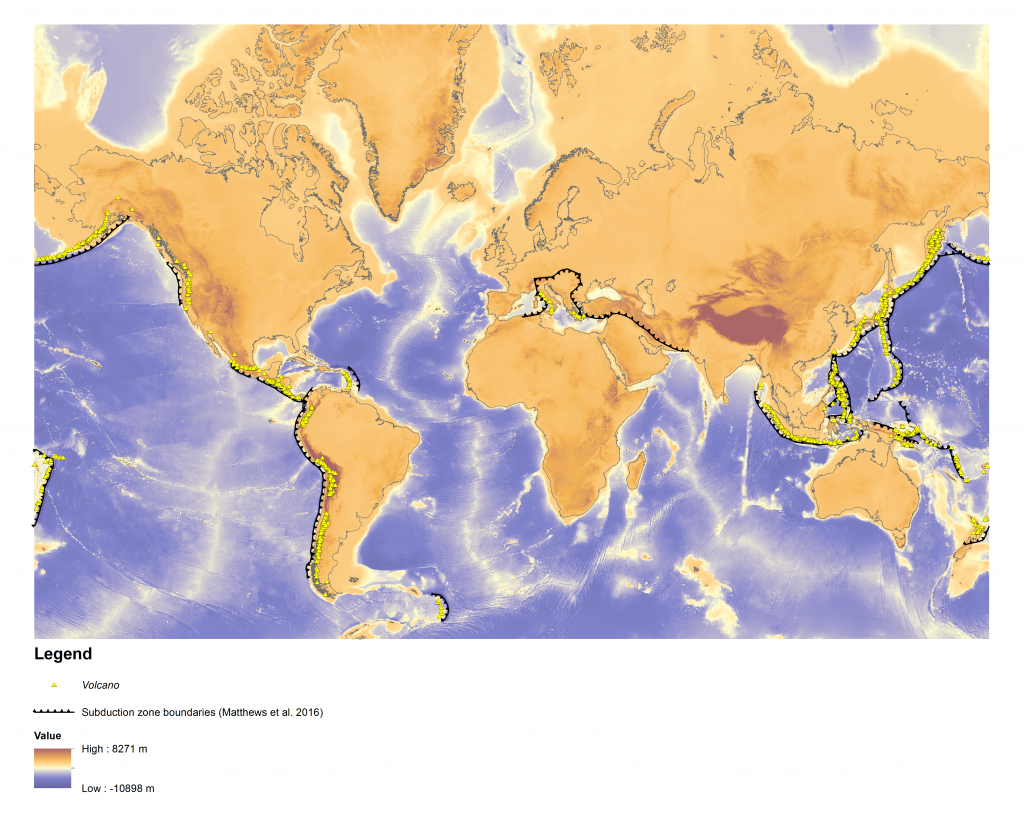# Calculating arc-trench distances using the Smithsonian Global Volcanism Project databaseFigure 1. Using ArcMap to visualise and calculate the distances between volcano locations (data points, yellow triangles) and subduction zones (black polylines).

Based on a study of 20 selected segments of arc-trench systems, Dickson (1973) found that the distance between trench axes to magmatic arc axes (or volcanic front) ranged from between 100 to 300 km in width.

Using the ‘Volcanoes of the World’ database maintained by the Smithsonian Institution’s Global Volcanism Program, we filtered the database for all volcanoes (both active and extinct) found in a subduction setting (in thick and intermediate continental crust and in thin, oceanic crust) and exported it as an Excel file. After extracting the latitudes and longitudes, we exported the data in a CSV file format to be read by ArcGIS.

We extracted subduction zone geometries at 0 Ma from our subduction zone analysis script and uploaded it in ArcGIS. It was automatically projected in WGS84 format, so we re-projected it using the ‘Project (Data Management)’ tool into World Mercator format (Figure 1).

We converted the CSV file of the volcano coordinates into X-Y points, and introduced it as a new layer in ArcMap. Again, we re-projected it using the ‘Project’ tool so that both the subduction zone lengths and the volcano data points had distances measured in metres and not degrees.

Finally we used the ‘Near (Analysis)’ tool to calculate the shortest distances between subduction zone trenches and volcanoes to represent the trench-to-arc gap. We exported the resulting attribute table to Excel and after running a basic statistical analysis we calculated that the average trench-to-arc distances are 286 km (Table 1). Statistically, the majority of volcanoes will lie within a distance of subduction zones equivalent to the mean + standard deviation, which was 448 km.

Thus, in our analysis that calculated the intersection of volcanic arcs with carbonate platforms, the maximum distance we used to search for carbonate platforms from the subduction zone boundaries was 448 km (Table 1). This part of the analysis is reproduced in a post here.

 Table 1. Distance of Volcano from Subduction zone trench Mean 287 km Median 281 km Minimum value 2 km Maximum value 1539 km Mode 181 km Standard deviation 161 km Mean + standard deviation 448 km